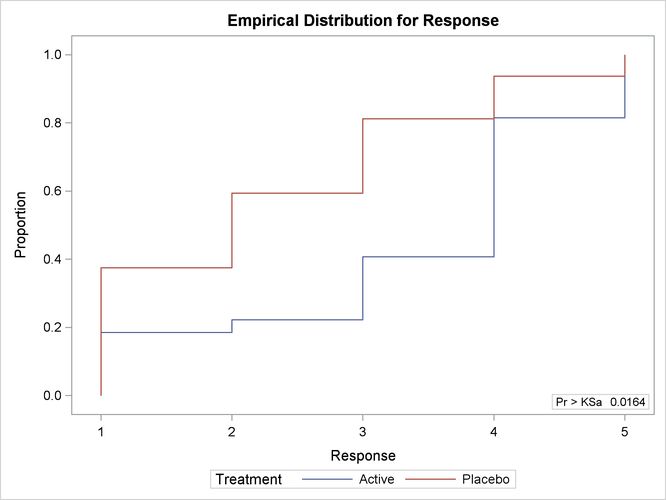# The NPAR1WAY Procedure

### Example 65.2 EDF Statistics and EDF Plot

This example uses the SAS data set `Arthritis` created in Example 65.1. The data set contains the variable `Treatment`, which denotes the treatment received by a patient, and the variable `Response`, which contains the response status of the patient. The variable `Freq` contains the frequency of the observation, which is the number of patients with the `Treatment` and `Response` combination.

The following statements request empirical distribution function (EDF) statistics, which test whether the distribution of a variable is the same across different groups. The EDF option requests the EDF analysis. The variable `Treatment` is the CLASS variable, and the variable `Response` specified in the VAR statement is the analysis variable. The FREQ statement names `Freq` as the frequency variable.

The PLOTS= option requests an EDF plot for `Response` classified by `Treatment`. ODS Graphics must be enabled before producing plots.

```ods graphics on;
proc npar1way edf plots=edfplot data=Arthritis;
class Treatment;
var Response;
freq Freq;
run;
ods graphics off;
```

Output 65.2.1 shows EDF statistics that compare the two levels of `Treatment`, Active and Placebo. The asymptotic p-value for the Kolmogorov-Smirnov test is 0.0164. This supports rejection of the null hypothesis that the distributions are the same for the two samples.

Output 65.2.2 shows the EDF plot for `Response` classified by `Treatment`.

Output 65.2.1: Empirical Distribution Function Statistics

The NPAR1WAY Procedure

Kolmogorov-Smirnov Test for Variable Response
Classified by Variable Treatment
Treatment N EDF at
Maximum
Deviation from Mean
at Maximum
Active 27 0.407407 -1.141653
Placebo 32 0.812500 1.048675
Total 59 0.627119

Kolmogorov-Smirnov Two-Sample Test
(Asymptotic)
KS 0.201818 D 0.405093
KSa 1.550191 Pr > KSa 0.0164

Output 65.2.2: Empirical Distribution Function Plot